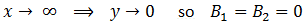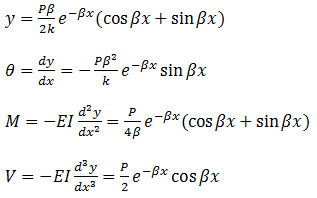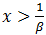top of page

#### Governed Differential Equation for a Beam on an Elastic Foundation

The resulting equations from this model are based on some discontinuity stress calculations. At this model an infinite beam which is under a lateral force (as shown in figure 1) is laid on an elastic foundation developing a uniform supporting load per unit length of the beam. The model intentions are as follows:

• Shear force and bending moment calculation in discontinuity area

• Deflection and rotation of points on the beam in discontinuity area

• #### Discontinuity area dimensions (Width)

First item helps us to calculate discontinuity stresses and effective discontinuity area where its effect is considerable.Figure 1: Model of infinite beam on an elastic foundation(1)

(2)

(3)

From (2) and (3):(4)

The linear differential equation for deflection of a beam due to pure bending moment is:(5)(6)

With (4) and (6):(7)

is a possible solution of equation (7). Therefore,(8)(9)

(10)is defined as character factor, β, so,(11)

The four solutions of equation (7) are as follows:So general solution of equation (7) is:(13)

Shear force and bending moment can be calculated by equations (1) and (5).Values of               to  depend on the model on which study is conducted and are determined by applying the model boundary conditions. For an infinite beam on an elastic foundation with concentrated load, boundary conditions are described below:1-

(14)

(12)

Due to a symmetric loading and geometry, the slope of the beam at the concentrated load point is zero. So:2-

3-

(15)

(16)

With equations (13), (14) and (15):(17)

By substituting equations (13) to (17) in equation (12), deflection of the beam can be achieved by the following equation (18). Moreover slope, bending moment and shear force being as a function of x are calculated by equations (19), (20) and (21) respectively.(18)

(19)

(20)

(21)

Those variables                 can be drawn versus  as shown on Fig. 5.3 of .The diagram demonstrates a significant reduction in shear stress and bending moment in the area where          . This reduction leads to a corresponding decrease in discontinuity stress beyond the discontinuity area which is limited to            from the concentrated load.bottom of page